# opencv6.4-imgproc图像处理模块之直方图与模板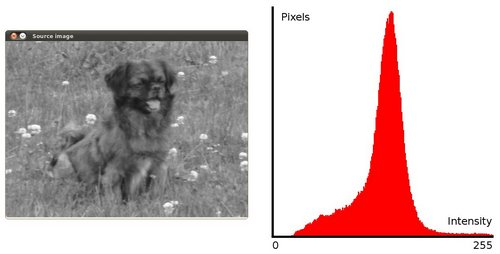1、均衡化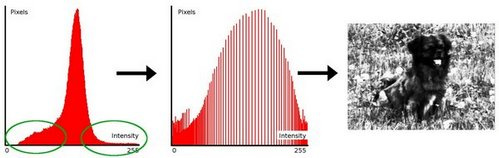均衡化指的是把一个分布 (给定的直方图) 映射 到另一个分布 (一个更宽更统一的强度值分布), 所以强度值分布会在整个范围内展开.

要想实现均衡化的效果, 映射函数应该是一个 累积分布函数 (cdf) (更多细节, 参考*学习OpenCV*). 对于直方图H(i), 它的 累积分布H'(i)是: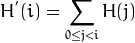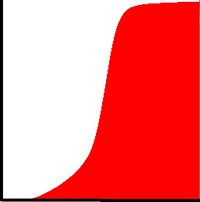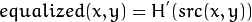/// 转为灰度图
cvtColor( src, src, CV_BGR2GRAY );

/// 应用直方图均衡化
equalizeHist( src, dst );

note：该函数是归一化图像的亮度和增加对比度。

2、计算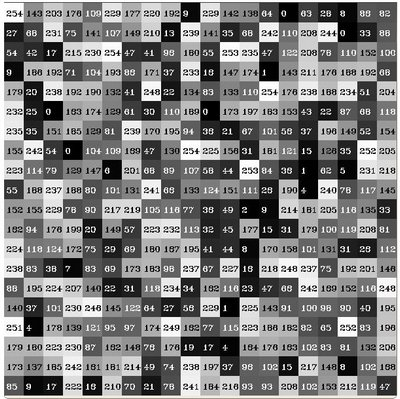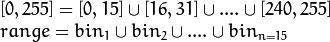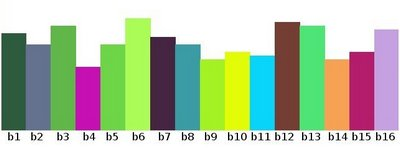以上只是一个说明直方图如何工作以及它的用处的简单示例。直方图可以统计的不仅仅是颜色灰度， 它可以统计任何图像特征 (如 梯度, 方向等等)。

dims: 需要统计的特征的数目， 在上例中， dims = 1 因为我们仅仅统计了灰度值(灰度图像)。

bins: 每个特征空间 子区段 的数目，在上例中, bins = 16

range: 每个特征空间的取值范围，在上例中， range = [0,255]

怎样去统计两个特征呢? 在这种情况下， 直方图就是3维的了，x轴和y轴分别代表一个特征， z轴是掉入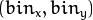组合中的样本数目。 同样的方法适用于更高维的情形 (当然会变得很复杂)。OpenCV提供了一个简单的计算数组集(通常是图像或分割后的通道)的直方图函数 calcHist 。 支持高达 32 维的直方图。

/// 分割成3个单通道图像 ( R, G 和 B )
vector<Mat> rgb_planes;
split( src, rgb_planes );

/// 设定bin数目
int histSize = 255;//这里是一个bin占了一个像素

/// 设定取值范围 ( R,G,B) )
float range[] = { 0, 255 } ;//这是RGB模型，不是HSV等模型。
const float* histRange = { range };//范围数组

bool uniform = true; bool accumulate = false;//把bin范围设定成同样大小(均一)；以及开始统计前先清除直方图中的痕迹

Mat r_hist, g_hist, b_hist;//创建储存R、G、B三个通道的直方图矩阵

/// 计算直方图:
calcHist( &rgb_planes, 1, 0, Mat(), r_hist, 1, &histSize, &histRange, uniform, accumulate );
calcHist( &rgb_planes, 1, 0, Mat(), g_hist, 1, &histSize, &histRange, uniform, accumulate );
calcHist( &rgb_planes, 1, 0, Mat(), b_hist, 1, &histSize, &histRange, uniform, accumulate );

// 创建直方图画布
int hist_w = 400; int hist_h = 400;
int bin_w = cvRound( (double) hist_w/histSize );

Mat histImage( hist_w, hist_h, CV_8UC3, Scalar( 0,0,0) );//显示不同直方图的画布

/// 将直方图归一化到范围 [ 0, histImage.rows ]，用 normalize 归一化直方图，这样直方图bin中的值就被缩放到指定范围
//归一化的原因在于之前的calcHist是归一化每个bin的范围，而这里是为了让两幅直方图图能够在同一个画布上显示
normalize(r_hist, r_hist, 0, histImage.rows, NORM_MINMAX, -1, Mat() );
normalize(g_hist, g_hist, 0, histImage.rows, NORM_MINMAX, -1, Mat() );
normalize(b_hist, b_hist, 0, histImage.rows, NORM_MINMAX, -1, Mat() );

/// 在直方图画布上画出直方图

r_hist.at<float>(i):i 指示维度，假如我们要访问2维直方图，我们就要用到这样的表达式:   r_hist.at<float>( i, j )

for( int i = 1; i < histSize; i++ )   {
line( histImage, Point( bin_w*(i-1), hist_h - cvRound(r_hist.at<float>(i-1)) ) ,//上一个末端作为当前的起始端
Point( bin_w*(i), hist_h - cvRound(r_hist.at<float>(i)) ),//当前所需要的末端，作为下一个的起始端
Scalar( 0, 0, 255), 2, 8, 0  );//颜色及线条的粗度等信息
line( histImage, Point( bin_w*(i-1), hist_h - cvRound(g_hist.at<float>(i-1)) ) ,
Point( bin_w*(i), hist_h - cvRound(g_hist.at<float>(i)) ),
Scalar( 0, 255, 0), 2, 8, 0  );
line( histImage, Point( bin_w*(i-1), hist_h - cvRound(b_hist.at<float>(i-1)) ) ,
Point( bin_w*(i), hist_h - cvRound(b_hist.at<float>(i)) ),
Scalar( 255, 0, 0), 2, 8, 0  );    } /// 显示直方图
namedWindow("calcHist Demo", CV_WINDOW_AUTOSIZE );
imshow("calcHist Demo", histImage );

hist, int dims, const int* histSize, const float** ranges, bool uniform=true, bool accumulate= false );

cvtColor(src, hsv, COLOR_BGR2HSV);

int hbins = 30, sbins = 32;// Quantize the hue to 30 levels and the saturation to 32 levels
int histSize[] = {hbins, sbins};
float hranges[] = { 0, 180 };// hue varies from 0 to 179, see cvtColor
float sranges[] = { 0, 256 };
const float* ranges[] = { hranges, sranges };
MatND hist;//typedef Mat MatND;同一个东西罢了
int channels[] = {0, 1};
// we compute the histogram from the 0-th and 1-st channels
calcHist( &hsv, 1, channels, Mat(), // do not use mask
hist, 2, histSize, ranges,
true, // the histogram is uniform
false );
double maxVal=0;
minMaxLoc(hist, 0, &maxVal, 0, 0);//留待以后介绍？？？？
int scale = 10;
Mat histImg = Mat::zeros(sbins*scale, hbins*10, CV_8UC3);
for( int h = 0; h < hbins; h++ )
for( int s = 0; s < sbins; s++ )
{
float binVal = hist.at<float>(h, s);
int intensity = cvRound(binVal*255/maxVal);
rectangle( histImg, Point(h*scale, s*scale),
Point( (h+1)*scale - 1, (s+1)*scale - 1),
Scalar::all(intensity),
CV_FILLED );
}
namedWindow( "Source", 1 );
imshow( "Source", src );
namedWindow( "H-S Histogram", 1 );
imshow( "H-S Histogram", histImg );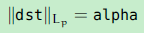；或者当normTpye=NORM_MINMXA(只对密集数组有用)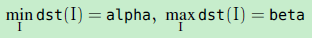。可选的掩码指定一个子数组进行归一化。也就是说norm或者min-n-max可以基于这个子数组计算得到，然后这个子数组来被归一化。如果只想计算这个norm或者min-max 但是却想修改整个数组，你可以使用norm（）和MAT::convertTo()。

3、对比

OpenCV 函数 compareHist 执行了具体的直方图对比的任务。该函数提供了好几种对比标准来计算相似度，不同版本的个数不同，在3.0beta中提供了6种，在2.49版本中提供了5种：

a、Correlation ( CV_COMP_CORREL )：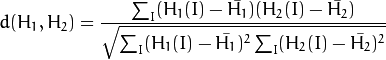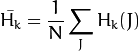N是直方图中bin的数目。

b、Chi-Square ( CV_COMP_CHISQR )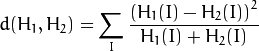c、Alternative Chi-Square (method=CV_COMP_CHISQR_ALT)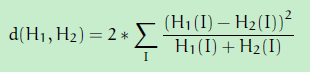这个 alternative 公式 通常用来作为纹理对比

c、Intersection ( CV_COMP_INTERSECT )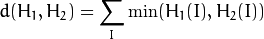d、Bhattacharyya 距离( CV_COMP_BHATTACHARYYA或者CV_COMP_HELLINGER )，在opencvn中计算的hellinger距离是与             bhattacharyya系数相关的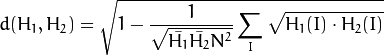e、Kullback-Leibler divergence (method=CV_COMP_KL_DIV).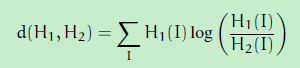Mat src_base, hsv_base;//声明原图像和转换到hsv的图像
Mat hsv_half_down;//提取转换后hsv下半部分的图像
cvtColor( src_base, hsv_base, CV_BGR2HSV );//rgb转换到hsv空间
hsv_half_down = hsv_base( Range( hsv_base.rows/2, hsv_base.rows - 1 ), Range( 0, hsv_base.cols - 1 ) );//提取下半部分
/// 对hue通道使用30个bin,对saturatoin通道使用32个bin
int h_bins = 50; int s_bins = 60;
int histSize[] = { h_bins, s_bins };

// hue的取值范围从0到256, saturation取值范围从0到180
float h_ranges[] = { 0, 256 };
float s_ranges[] = { 0, 180 };

const float* ranges[] = { h_ranges, s_ranges };

// 使用第0和第1通道
int channels[] = { 0, 1 };

/// 直方图 其实MatND就等于Mat
MatND hist_base;
MatND hist_half_down;
/// 计算HSV图像的直方图
calcHist( &hsv_base, 1, channels, Mat(), hist_base, 2, histSize, ranges, true, false );
normalize( hist_base, hist_base, 0, 1, NORM_MINMAX, -1, Mat() );

calcHist( &hsv_half_down, 1, channels, Mat(), hist_half_down, 2, histSize, ranges, true, false );
normalize( hist_half_down, hist_half_down, 0, 1, NORM_MINMAX, -1, Mat() );

///应用不同的直方图对比方法
for( int i = 0; i < 4; i++ )
{ int compare_method = i;
double base_base = compareHist( hist_base, hist_base, compare_method );
double base_half = compareHist( hist_base, hist_half_down, compare_method );

}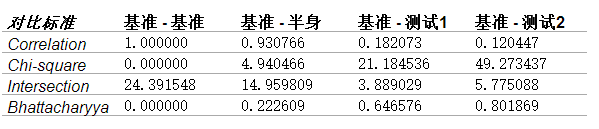（最后两列不再代码里面，这个是为了说明四种标准的评判趋势的，）

range函数原型

class CV_EXPORTS Range{public:     Range();     Range(int _start, int _end);     Range(const CvSlice& slice);     int size() const;     bool empty() const;     static Range all();     operator CvSlice() const;     int start, end;};


void my_function(..., const Range& r, ....)
{
if(r == Range::all()) {
// process all the data
}
else {
// process [r.start, r.end)
}
}


double compareHist(const SparseMat& H1, const SparseMat& H2, int method)

– CV_COMP_CORREL Correlation
– CV_COMP_CHISQR Chi-Square
– CV_COMP_CHISQR_ALT Alternative Chi-Square （来自3.0beta）
– CV_COMP_INTERSECT Intersection
– CV_COMP_BHATTACHARYYA Bhattacharyya distance
– CV_COMP_HELLINGER Synonym for CV_COMP_BHATTACHARYYA
– CV_COMP_KL_DIV Kullback-Leibler divergence； （来自3.0beta）

4、反向投影

a、假设你已经通过下图得到一个肤色直方图(Hue-Saturation)， 旁边的直方图就是 模型直方图 ( 代表手掌的皮肤色调).你可以通过掩码操作来抓取手掌所在区域的直方图: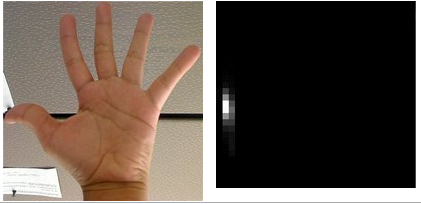b、下图是另一张手掌图(测试图像) 以及对应的整张图像的直方图：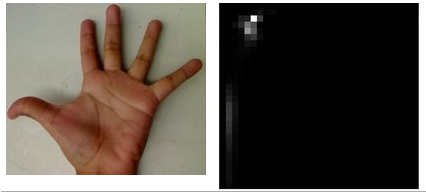c、我们要做的就是使用 模型直方图 (代表手掌的皮肤色调) 来检测测试图像中的皮肤区域。以下是检测的步骤：

i）对测试图像中的每个像素 p(i,j),获取色调数据并找到该色调(h_ij,s_ij)在直方图中的bin的位置。

ii）查询 模型直方图 中对应的bin -（h_ij,s_ij） - 并读取该bin的数值。

iii）将此数值储存在新的图像中(BackProjection)。 你也可以先归一化 模型直方图 ,这样测试图像的输出就可以在屏幕显示了。

iiii)通过对测试图像中的每个像素采用以上步骤， 我们得到了下面的 BackProjection 结果图: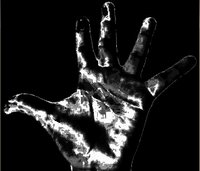iiiii）使用统计学的语言, BackProjection 中储存的数值代表了测试图像中该像素属于皮肤区域的 概率 。比如以上图为例， 亮起的区域是皮肤区域的概率更大(事实确实如此),而更暗的区域则表示更低的概率(注意手掌内部和边缘的阴影影响了检测的精度)。

。这里的一个保证是之前提取的模型是对的，那么假设其颜色区域的直方图范围为200-230之间的直方图的bin，然后读取的当前像素位置的值在去查找模型的值，模型中那个对应的bin是20，也就是有20个像素点落在这里，然后在测试图像刚刚那个像素点位置上填上20，所以结果就是不再模型中的直方图。例如灰度或者颜色的部分就被忽略了。乍一看是很好，不过觉得这个鲁棒性肯定很差。理解这个的突破点在于最后得到的那个backprojection是个统计矩阵。相比较背景来说，颜色不同亮度不同所以可以被忽略。

/// 全局变量
Mat src; Mat hsv; Mat hue;
int bins = 25;
 /// 转换到 HSV 空间
cvtColor( src, hsv, CV_BGR2HSV );
/// 分离 Hue 通道
hue.create( hsv.size(), hsv.depth() );//创建个空值矩阵
int ch[] = { 0, 0 };
mixChannels( &hsv, 1, &hue, 1, ch, 1 );//抽取 HSV图像的0通道(Hue)
  MatND hist;
int histSize = MAX( bins, 2 );//#  define MAX(a,b)  ((a) < (b) ? (b) : (a))
float hue_range[] = { 0, 180 };
const float* ranges = { hue_range };

/// 计算直方图并归一化
calcHist( &hue, 1, 0, Mat(), hist, 1, &histSize, &ranges, true, false );
normalize( hist, hist, 0, 255, NORM_MINMAX, -1, Mat() );

/// 计算反向投影
MatND backproj;
calcBackProject( &hue, 1, 0, hist, backproj, &ranges, 1, true );

/// 显示反向投影
imshow( "BackProj", backproj );

/// 显示直方图
int w = 400; int h = 400;
int bin_w = cvRound( (double) w / histSize );
Mat histImg = Mat::zeros( w, h, CV_8UC3 );

for( int i = 0; i < bins; i ++ )
{ rectangle( histImg, 
                 Point( i*bin_w, h ),
                 Point( (i+1)*bin_w, h - cvRound( hist.at<float>(i)*h/255.0 ) ),
                 Scalar( 0, 0, 255 ), -1 ); }

imshow( "Histogram", histImg );
}

void mixChannels(InputArrayOfArrays src, InputOutputArrayOfArrays dst, const int* fromTo, size_t npairs)；
void mixChannels(InputArrayOfArrays src, InputOutputArrayOfArrays dst, const std::vector<int>&fromTo)；

nsrcs：在src中的矩阵的个数；

dst：输出的数组或者是矩阵向量；所有的矩阵必须已经被预分配了空间；他们的size和depth必须和src【0】中的一致

ndst：在dst中的矩阵的个数；

fromTo：索引对的数组，用来指定哪个通道被复制和复制到哪里；fromTo[k*2]在src中是一个基于0（以0作为开始）的输入通道的索引；fromTo[k*2+1]在dst中是作为输出通道的索引；可以使用连续的通道数字；第一个输入图像的通道由0到src【0】.channels（）-1,第二个输入图像的通道由src【0】.channels（）到src【0】.channels（）+src【1】.channels（）-1，以此类推。同样的方案可以用来指定输出图像的通道；作为一个特别的例子，当fromTo[k*2]是负的时候，对应的输出通道可以用0来填充。

npairs：在fromTo中索引对的个数。

Mat rgba( 100, 100, CV_8UC4, Scalar(1,2,3,4) );
Mat bgr( rgba.rows, rgba.cols, CV_8UC3 );
Mat alpha( rgba.rows, rgba.cols, CV_8UC1 );

// forming an array of matrices is a quite efficient operation,
// because the matrix data is not copied, only the headers
Mat out[] = { bgr, alpha };
// rgba -> bgr, rgba -> bgr,
// rgba -> bgr, rgba -> alpha
int from_to[] = { 0,2, 1,1, 2,0, 3,3 };
mixChannels( &rgba, 1, out, 2, from_to, 4 );；


void calcBackProject(const Mat* images, int nimages, const int* channels, const SparseMat& hist, OutputArray backProject, const float** ranges, double scale=1, bool uniform= true )；

nimages：原图像的个数；

channels：通道列表，用来计算反向投影的。通道数必须匹配直方图的维度数。第一个数组通道由0到images.channels()-1，第二个数组通道         由images.channels()到images.channels()+images.channels()-1，以此类推；

hist：输入的直方图，可以是密集或者稀疏的；

backProject：目标反向投影数组，必须是和images一样size和depth的单通道数组；

ranges：每个维度上直方图bin边界数组的数组，可见calcHist()；

scale：可选的为了输出反向投影的缩放因子；

uniform：标识，用来指示直方图是否需要归一化。

a、在追踪之前，将对象进行拍照并且使得对象几乎能够占满整个图像帧。计算hue直方图。这个直方图会有很强的最大值，对应着对象中的领域颜色；

b、在追踪的时候，使用预计算过的直方图来计算每个输入视频帧的hue平面的反向投影。使用阈值反向索引来压缩弱颜色。对于没有足够的颜色饱和度的压缩像素和太黑或者太亮的像素来说都是很有意义的。

c、查找相连的成分生成图像，并选定最大的成分。

原图像 (I): 在这幅图像里,我们希望找到一块和模板匹配的区域

模板 (T): 将和原图像比照的图像块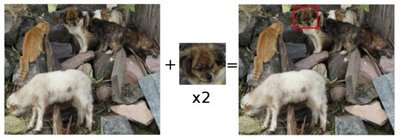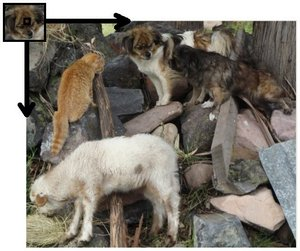通过 滑动, 我们的意思是图像块一次移动一个像素 (从左往右,从上往下). 在每一个位置, 都进行一次度量计算来表明它是 “好” 或 “坏” 地与那个位置匹配 (或者说块图像和原图像的特定区域有多么相似).

对于 T 覆盖在 I 上的每个位置,你把度量值 保存 到 结果图像矩阵 (R) 中. 在 R 中的每个位置（x，y）都包含匹配度量值: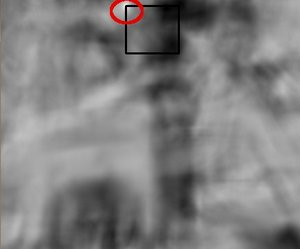上图就是 TM_CCORR_NORMED 方法处理后的结果图像 R . 最白的位置代表最高的匹配. 正如您所见, 红色椭圆框住的位置很可能是结果图像矩阵中的最大数值, 所以这个区域 (以这个点为顶点,长宽和模板图像一样大小的矩阵) 被认为是匹配的.

实际上, 我们使用函数 minMaxLoc 来定位在矩阵 R 中的最大值点 (或者最小值, 根据函数输入的匹配参数) .

OpenCV通过函数 matchTemplate 实现了模板匹配算法. 可用的方法有6个:

下面的符号：I表示image，T表示template，R表示result。得到的结果为x' = 0,...，w-1；y' = 0，...，h-1。

a、平方差匹配 method=CV_TM_SQDIFF：这类方法利用平方差来进行匹配,最好匹配为0.匹配越差,匹配值越大.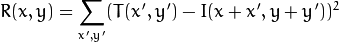b、标准平方差匹配 method=CV_TM_SQDIFF_NORMED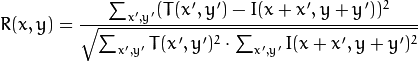c、相关匹配 method=CV_TM_CCORR：这类方法采用模板和图像间的乘法操作,所以较大的数表示匹配程度较高,0标识最坏的匹配效果.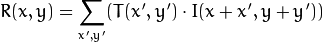d、标准相关匹配 method=CV_TM_CCORR_NORMED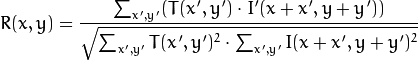e、相关匹配 method=CV_TM_CCOEFF：这类方法将模版对其均值的相对值与图像对其均值的相关值进行匹配,1表示完美匹配,-1表示糟糕的匹配,0表示没有任何相关性(随机序列).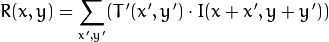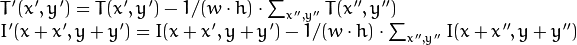f、标准相关匹配 method=CV_TM_CCOEFF_NORMED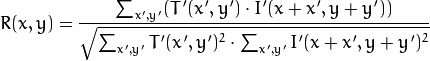通常,随着从简单的测量(平方差)到更复杂的测量(相关系数),我们可获得越来越准确的匹配(同时也意味着越来越大的计算代价). 最好的办法是对所有这些设置多做一些测试实验,以便为自己的应用选择同时兼顾速度和精度的最佳方案.

在该函数完成对比之后，最好的匹配可以使用函数minMaxLoc()查找全局最小值（CV_TM_SQDIFF）或者最大值（CV_TM_CCORR、CV_TM_CCOEFF）。在一个颜色图像中，基于所有的通道计算分子中的模板总和和分母中每个和，不过对于每个通道使用的是分离的均值。也就是说，这个函数可以采用一个颜色模板和一个彩色图像。这个结果仍然是一个单通道图像，这也更容易理解。

/// 载入原图像和模板块
templ = imread( );
/// 将被显示的原图像
Mat img_display;
img.copyTo( img_display );

/// 创建输出结果的矩阵，用来输出匹配结果，这里很像cnn中的卷积层中的特征子图
int result_cols =  img.cols - templ.cols + 1;
int result_rows = img.rows - templ.rows + 1;

result.create( result_cols, result_rows, CV_32FC1 );

/// 进行匹配和标准化
matchTemplate( img, templ, result, match_method );
normalize( result, result, 0, 1, NORM_MINMAX, -1, Mat() );

/// 通过函数 minMaxLoc 定位最匹配的位置
double minVal; double maxVal; Point minLoc; Point maxLoc;
Point matchLoc;

minMaxLoc( result, &minVal, &maxVal, &minLoc, &maxLoc, Mat() );

/// 对于方法 SQDIFF 和 SQDIFF_NORMED, 越小的数值代表更高的匹配结果. 而对于其他方法, 数值越大匹配越好
if( match_method  == CV_TM_SQDIFF || match_method == CV_TM_SQDIFF_NORMED )
{ matchLoc = minLoc; }
else
{ matchLoc = maxLoc; }

/// 让我看看您的最终结果
rectangle( img_display, matchLoc, Point( matchLoc.x + templ.cols , matchLoc.y + templ.rows ), Scalar::all(0), 2, 8, 0 );
rectangle( result, matchLoc, Point( matchLoc.x + templ.cols , matchLoc.y + templ.rows ), Scalar::all(0), 2, 8, 0 );

imshow( image_window, img_display );
imshow( result_window, result );

{
CV_TM_SQDIFF        =0,
CV_TM_SQDIFF_NORMED =1,
CV_TM_CCORR         =2,
CV_TM_CCORR_NORMED  =3,
CV_TM_CCOEFF        =4,
CV_TM_CCOEFF_NORMED =5
};

void minMaxLoc(const SparseMat& a, double* minVal, double* maxVal, int* minIdx=0, int* maxIdx=0 )；

minVal：指向返回的最小值的指针；当不需要的时候赋值NULL即可；

maxVal：指向返回的最大值的指针；当不需要的时候赋值NULL即可；

minLoc：指向返回的最小值的位置（在2D情况中）；当不需要的时候赋值NULL即可；

maxLoc：指向返回的最大值的位置（在2D情况中）；当不需要的时候赋值NULL即可；

04-222498

07-1488
06-26388
04-19438
09-112040
05-17386
11-189202
08-12730
08-17116
11-0290
01-27624
10-231万+
08-031413
01-291万+
05-093386
05-102201
11-241832
04-023023

### “相关推荐”对你有帮助么？

•非常没帮助
•没帮助
•一般
•有帮助
•非常有帮助¥2 ¥4 ¥6 ¥10 ¥20余额支付 (余额：-- )扫码支付获取中扫码支付点击重新获取扫码支付1.余额是钱包充值的虚拟货币，按照1:1的比例进行支付金额的抵扣。
2.余额无法直接购买下载，可以购买VIP、C币套餐、付费专栏及课程。余额充值